# WO2015122261A1 - Imaging lens and imaging device - Google Patents

Imaging lens and imaging device Download PDF

## Info

Publication number
WO2015122261A1
WO2015122261A1 PCT/JP2015/051969 JP2015051969W WO2015122261A1 WO 2015122261 A1 WO2015122261 A1 WO 2015122261A1 JP 2015051969 W JP2015051969 W JP 2015051969W WO 2015122261 A1 WO2015122261 A1 WO 2015122261A1
Authority
WO
WIPO (PCT)
Prior art keywords
lens
imaging
lt
image
imaging lens
Prior art date
Application number
PCT/JP2015/051969
Other languages
French (fr)
Japanese (ja)
Inventor

Original Assignee
コニカミノルタ株式会社
Priority date (The priority date is an assumption and is not a legal conclusion. Google has not performed a legal analysis and makes no representation as to the accuracy of the date listed.)
Filing date
Publication date
Priority to JP2014025395 priority Critical
Priority to JP2014-025395 priority
Application filed by コニカミノルタ株式会社 filed Critical コニカミノルタ株式会社
Publication of WO2015122261A1 publication Critical patent/WO2015122261A1/en

## Images

•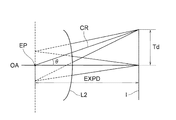•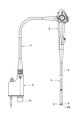•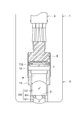•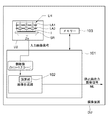•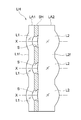•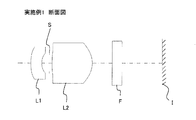•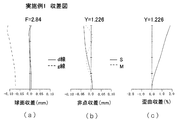•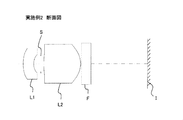•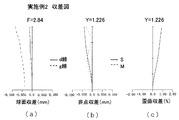••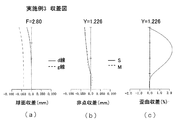•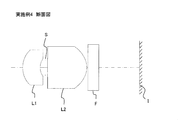•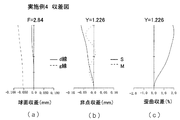•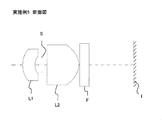•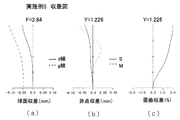•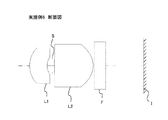•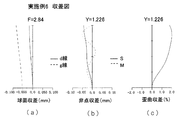•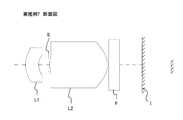•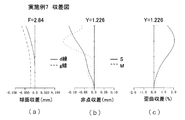•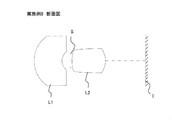•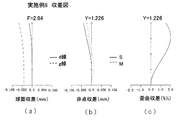•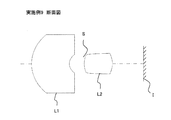•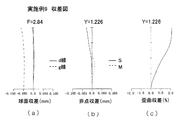•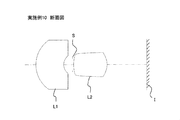•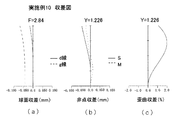••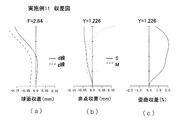•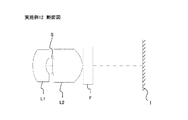•## Classifications

• AHUMAN NECESSITIES
• A61MEDICAL OR VETERINARY SCIENCE; HYGIENE
• A61BDIAGNOSIS; SURGERY; IDENTIFICATION
• A61B1/00Instruments for performing medical examinations of the interior of cavities or tubes of the body by visual or photographical inspection, e.g. endoscopes; Illuminating arrangements therefor
• A61B1/00064Constructional details of the endoscope body
• A61B1/00071Insertion part of the endoscope body
• A61B1/0008Insertion part of the endoscope body characterised by distal tip features
• A61B1/00096Optical elements
• AHUMAN NECESSITIES
• A61MEDICAL OR VETERINARY SCIENCE; HYGIENE
• A61BDIAGNOSIS; SURGERY; IDENTIFICATION
• A61B1/00Instruments for performing medical examinations of the interior of cavities or tubes of the body by visual or photographical inspection, e.g. endoscopes; Illuminating arrangements therefor
• A61B1/04Instruments for performing medical examinations of the interior of cavities or tubes of the body by visual or photographical inspection, e.g. endoscopes; Illuminating arrangements therefor combined with photographic or television appliances
• A61B1/05Instruments for performing medical examinations of the interior of cavities or tubes of the body by visual or photographical inspection, e.g. endoscopes; Illuminating arrangements therefor combined with photographic or television appliances characterised by the image sensor, e.g. camera, being in the distal end portion
• A61B1/051Details of CCD assembly
• GPHYSICS
• G02OPTICS
• G02BOPTICAL ELEMENTS, SYSTEMS, OR APPARATUS
• G02B23/00Telescopes, e.g. binoculars; Periscopes; Instruments for viewing the inside of hollow bodies; Viewfinders; Optical aiming or sighting devices
• G02B23/24Instruments or systems for viewing the inside of hollow bodies, e.g. fibrescopes
• G02B23/2407Optical details
• G02B23/2423Optical details of the distal end
• G02B23/243Objectives for endoscopes
• GPHYSICS
• G02OPTICS
• G02BOPTICAL ELEMENTS, SYSTEMS, OR APPARATUS
• G02B23/00Telescopes, e.g. binoculars; Periscopes; Instruments for viewing the inside of hollow bodies; Viewfinders; Optical aiming or sighting devices
• G02B23/24Instruments or systems for viewing the inside of hollow bodies, e.g. fibrescopes
• G02B23/2476Non-optical details, e.g. housings, mountings, supports
• G02B23/2484Arrangements in relation to a camera or imaging device

## Abstract

Provided is an imaging lens which has a two-lens structure, minimizes the angle of incidence of the principal ray onto the imaging surface periphery and favorably corrects various aberrations while allowing a considerably more compactness in the radial direction than conventional imaging lenses; also provided is an imaging device. This imaging lens comprises, in order from the object side, a first lens with a negative refractive power and a concave surface facing the image side, an aperture stop, and a second lens with a positive refractive power and a convex surface facing the image side, and satisfies the following conditions. 0.74 < D3/f < 1.50 (1) 0.68 < ΦLmax/2Yd < 1.70 (2) Here, D3: the thickness on the optical axis of the second lens f: the focal distance of the entire imaging lens system ΦLmax: the effective diameter of the lens with the greatest effective diameter Yd: the maximum image height

## Description

An imaging lens and an imaging apparatus

The present invention relates to an imaging apparatus using a solid-state image pickup element such as CCD type image sensor or a CMOS type image sensor, and an imaging lens for use therein.

Use in recent years, performance of CCD (Charge Coupled Device) type image sensor or CMOS (Complementary Metal Oxide Semiconductor) type image sensor of a solid-state image pickup device an image pickup device using, with the miniaturization, such solid-state imaging device There imaging apparatus, not only the portable terminal and the like, has come to be used in more various fields. Further, the imaging lens mounted in these image pickup apparatuses, there is an increasing demand for further miniaturization.

For example, a the imaging lens used built-in the imaging apparatus in medical endoscope to be inserted, particularly high demand for downsizing in the radial direction for the burden of the viewer to the body of the viewer there is a tendency that. Furthermore, with regard the solid-state imaging device, since it is possible to specially designed for the applications that are mass-produced as a portable terminal, if the principal ray incident angle to the outermost peripheral portion of the imaging surface is allowed up to about 30 ° contrast is located, in order to place a thin tube like a medical endoscope, there is a case forced using a general-purpose solid-state imaging device. In this case, it is necessary to design it to the principal ray incident angle is small suppressed imaging lens to the imaging surface in response. Furthermore, with regard the angle of view of the imaging lens, from the viewpoint that it is desired to observe an object in a wide range in a short distance, there is growing demand for a wide angle of the imaging lens.

In contrast, in order to achieve both a wide angle and miniaturization of the imaging lens, the first lens, two lenses of the imaging lens disposed in the order of the second lens having a positive refractive power discloses having a negative refractive power is (e.g., see Patent documents 1 and 2).

Pat. No. 3567109 JP 2001-174701 JP

However, the imaging lens described in Patent Document 1, the first lens is larger than that of the second lens, at the same time the first lens is larger than the imaging surface size. For such an image pickup lens, it becomes possible radial size when incorporated into the imaging device is determined by the effective diameter of the first lens, inevitably large in the radial direction of the imaging device.

Further, the imaging lens described in Patent Document 2, although is smaller than the effective diameter of the first lens and the second lens are both imaging surface size, the principal ray incident angle to the imaging plane peripheral portion 20 ° greater a large, undesirable may malfunction such as shading occurs in the case of using a general-purpose solid-state imaging device.

The present invention has been made in view of such a problem, while it is possible to sufficiently miniaturize the conventional type in the radial direction, the principal ray incident angle on the imaging surface peripheral portion is suppressed, various aberrations are satisfactorily corrected, and an object thereof is to provide an imaging lens and an image pickup apparatus of two configurations.

To achieve at least one of the above objects, an imaging lens that reflects one aspect of the present invention is an imaging lens for forming an object image on the photoelectric conversion portion of the solid-state imaging device, the object side more order,
A first lens having a concave surface on the image side has a negative refractive power,
Aperture stop,
A positive second lens with a convex surface facing to have image side refracting power and,
An image pickup lens satisfies the following conditional expression.
0.74 <D3 / f <1.50 (1)
0.68 <ΦLmax / 2Yd <1.70 (2)
However,
D3: the second lens thickness on the optical axis f: focal length of the imaging lens system FaiLmax: most large lens effective diameter effective diameter Yd: maximum image height

Strong in front of the second lens having a positive refractive power, arranged relatively refraction weak negative first lens having power, by a so-called retrofocus type image pickup lens, downsizing and a wide angle of the imaging lens length I have both. Further, an aperture stop that is disposed between the first lens and the second lens with respect to the aperture stop, because the first lens and the second lens can be a concentric arrangement, advantageous for aberration correction the Do configuration.

Condition (1), while performing aberration correction is a condition for obtaining a good image-side telecentric characteristics and size of the imaging lens. A value of the expression (1) exceeds the lower limit, it was reasonably free of thickness of the second lens, the refractive power of the second lens can be adequately secured, obtain a good image-side telecentric characteristics it can. If the thickness of the second lens is large, it means that the light beam for each image height passing through the second lens image side surface is passed through the different positions, it is advantageous for correction of field curvature. On the other hand, when a value of the expression (1) is lower than the upper limit, not too large thickness of the second lens than necessary, can be achieved a reduction in the size of the optical axis of the imaging lens.

Condition (2), with respect to the maximum image height of the image formed by the imaging lens, a conditional expression for appropriately setting the ratio of the effective diameter of the large lens of the most effective diameter. A value of the expression (2) is below the upper limit, the maximum image height of the image formed to not the lens diameter is too large, it is possible to reduce the size of the radial direction of the imaging device the entire system. On the other hand, when a value of the expression (2) exceeds the lower limit, it as the effective diameter of the imaging lens does not become too small for the solid-state imaging device. When the effective diameter of the imaging lens becomes smaller than the maximum image height, ray angle coming emitted from the imaging lens becomes larger correspondingly. Therefore, in order to obtain a good image-side telecentric characteristics, the effective diameter of the imaging lens is important not too small relative to the maximum image height.

The imaging lens may have a lens having substantially no refractive power. That is, the above-described configuration, it is within the scope of the present invention even when applying the lens having substantially no power.

This imaging apparatus comprising: the above image pickup lens, an image sensor which photoelectrically converts an image formed by the imaging lens.

In the imaging apparatus, the imaging lens is arranged in a direction orthogonal to the optical axis in an array, the imaging device can be configured to photoelectrically convert an image, each formed of the imaging lens.

According to the present invention, while it is possible to sufficiently miniaturize the conventional type in the radial direction, the principal ray incident angle on the imaging surface peripheral portion is suppressed, various aberrations are satisfactorily corrected, two lenses it is possible to provide an imaging lens and an imaging apparatus.

It is a diagram for explaining an example of the present invention. Is a diagram illustrating the overall configuration of an electronic endoscope having an imaging device according to the first embodiment. It is an enlarged sectional view of the distal optical unit portion for holding the objective optical system OS and the solid-state image sensor 8 in the distal end portion main body 5 of the electronic endoscope. It is a schematic diagram of an imaging apparatus using the compound-eye optical system according to the second embodiment. It is a cross-sectional view of the compound-eye optical system LH. An optical axis direction sectional view of the imaging lens of Example 1. Aberration diagram of Example 1 is (spherical aberration (a), astigmatism (b), distortion aberration (c)). An optical axis direction sectional view of the imaging lens of Example 2. Aberration diagram of Example 2 is (spherical aberration (a), astigmatism (b), distortion aberration (c)). An optical axis direction sectional view of the imaging lens of Example 3. Aberration diagram of Example 3 is (spherical aberration (a), astigmatism (b), distortion aberration (c)). An optical axis direction sectional view of the imaging lens of Example 4. Aberration diagram of Example 4 is (spherical aberration (a), astigmatism (b), distortion aberration (c)). An optical axis direction sectional view of the imaging lens of Example 5. Aberration diagram of Example 5 is a (spherical aberration (a), astigmatism (b), distortion aberration (c)). An optical axis direction sectional view of the imaging lens of Example 6. Aberration diagram of Example 6 is (spherical aberration (a), astigmatism (b), distortion aberration (c)). An optical axis direction sectional view of the imaging lens of Example 7. Aberration diagram of Example 7 is (spherical aberration (a), astigmatism (b), distortion aberration (c)). An optical axis direction sectional view of the imaging lens of Example 8. Aberration diagram of Example 8 is a (spherical aberration (a), astigmatism (b), distortion aberration (c)). An optical axis direction sectional view of the imaging lens of Example 9. Aberration diagram of Example 9 is (spherical aberration (a), astigmatism (b), distortion aberration (c)). An optical axis direction sectional view of the imaging lens of Example 10. Aberration diagram of Example 10 is a (spherical aberration (a), astigmatism (b), distortion aberration (c)). An optical axis direction sectional view of the imaging lens of Example 11. Aberration diagram of Example 11 is a (spherical aberration (a), astigmatism (b), distortion aberration (c)). An optical axis direction sectional view of the imaging lens of Example 12. Aberration diagram of Example 12 is a (spherical aberration (a), astigmatism (b), distortion aberration (c)).

Hereinafter, the embodiments of the present invention will be described with reference to the drawings. Figure 2 shows the overall configuration of an electronic endoscope having an imaging device according to the first embodiment. In Figure 2, the proximal end of the flexible insertion portion 1 operating unit 2 is connected, connected to the connector 3 connected to the video processor, not shown, extending from the plane upper part near the rear of the operating unit 2 Friendly It connected to the distal end of the Shiwakan 4.

The insertion portion 1 of the tip distal end portion main body 5 connected to the objective optical system OS of the imaging lens is disposed, CCD type image sensor on the image side, the imaging of the solid-state image pickup device 8 such as a CMOS image sensor surface is located. 9, the image signal output from the solid-state imaging device 8, a signal cable for transmitting the signal connector portion 10 disposed at the distal end of the connector 3.

Figure 3 shows the distal optical unit portion for holding the objective optical system OS and the solid-state image sensor 8 in the distal end portion main body 5 of the electronic endoscope, an imaging device holding the solid-state imaging device 8 is fixed to the inside cylinder 12, the distal end side (lower side in FIG. 3) is opened rear end is sealed airtight. I is the imaging surface of the solid-state imaging device 8, CG is a cover glass of the solid-state imaging device 8. Note between the objective optical system OS and a cover glass CG, various filters are arranged according to the application.

Lens holding barrel 13 holding the objective optical system OS, the rear end side portion is fitted and fixed to the inner periphery of the distal end side of the image sensor holding barrel 12 back end side (upper side in FIG. 3) is opened there. Objective optical system OS, in order from the object side, a first lens L1, an aperture stop provided in the light shielding member SH S, positive image side has a refractive power with a concave surface facing the image side has a negative refractive power and a second lens L2, the having a convex surface directed toward the. F is a mounting portion of various optical filters.

The distal end portion main body 5 of the electronic endoscope shown in FIG. 3 when inserted into the body of the observer, reflected light of the observed portion by a light source (not shown) is incident on the objective optical system OS, further solid-state imaging device It is converted into an electric signal by 8 image on the imaging surface I, the input to the signal connector 10 through a cable 9, so that the image of the observation area is displayed on an external monitor.

Next, the image pickup apparatus or the like according to the second embodiment. In the present embodiment, in which the imaging lens of the present invention is applied to a compound-eye optical system. Compound eye optical system includes a plurality of lens systems for one image sensor (ommatidium optical system) is an optical system arranged in an array, the type and, the lenses each lens system performs imaging of substantially the same field of view system is a field split type for imaging different fields of view, usually divided into. Compound-eye optical system according to the present invention can be used in any type, here oriented in the same direction, and a plurality of images obtained by a plurality of lens system having a small parallax, than the individual image is described type are also used in super-resolution processing for outputting one composite image with high resolution.

The imaging apparatus according to this embodiment is shown schematically in FIG. As shown in FIG. 4, the imaging apparatus DU includes an imaging unit LU, the image processing unit 101, calculation unit 102, a memory 103 or the like. The imaging unit LU has a single image sensor SR, for the image sensor SR, a compound-eye optical system LH for forming a plurality of images having a small parallax, the. The image sensor SR, for example a CCD image sensor having a plurality of pixels, the solid-state imaging device such as a CMOS image sensor is used. The photoelectric conversion unit in which the light receiving surface or on the imaging plane I of the image sensor SR, since the compound-eye optical system LH as optical image of an object is formed is provided, formed by the compound-eye optical system LH optical image is converted into an electrical signal by the image sensor SR, respectively. Image in the image synthesis unit in the processing unit 101, based on the electrical signal corresponding to a plurality of images sent from the image sensor SR by the operation unit 102, a higher resolution one image data from a plurality of images (Synthesis performing an image processing so as to obtain an image ML).

Figure 5 is a cross-sectional view of the compound-eye optical system LH. Towards the left of Figure 5 is the object side. Compound-eye optical system LH, in order from the object side, a plurality the object side lens L1 (here, the nine that arranged in three rows and three columns), a first flange portion L1f linking to each other object-side lens L1 is formed integrally an array lens LA1, and the light shielding member SH having an aperture stop S of a plurality (nine in which arranged in three rows and three columns in this case), the image side lens L2 of a plurality (nine in which arranged in three rows and three columns in this case) has a second array lens LA2 which flange L2f formed integrally connecting the image-side lens L2 each other, the. A first array lens LA1 second array lens LA2 is molded integrally with each polycarbonate, acrylic, or the like, are bonded by interposing the light shielding member SH. An object side lens L1 having a concave surface on a image side a negative refractive power, the optical axis X of the aperture stop S and a positive image-side lens a convex surface facing the image side has a refractive power L2 coincides and which, thereby constituting ommatidium optical system (imaging lens).

Compound-eye optical system LH is held in a lens frame (not shown), the lens frame is fixed to the substrate the image sensor SR is mounted.

A first lens L1 of FIG. 3, satisfying an aperture stop S, the following conditional expression composed imaging lens of the second lens L2 Prefecture (objective optical system OS) a (1) (2). The same applies in the compound eye optical system LH in FIG.

0.74 <D3 / f <1.50 (1)
0.68 <ΦLmax / 2Yd <1.70 (2)
However,
D3: the second lens thickness on the optical axis f: focal length of the imaging lens system FaiLmax: most large lens effective diameter effective diameter Yd: maximum image height

The imaging lens preferably satisfies the following conditional expression.
0.60 <f2 / f <1.10 (3)
However,
f2: the focal length f of the second lens focal length of the entire imaging lens system

Condition (3) is a conditional expression for setting the focal length of the second lens properly. A value of the expression (3) exceeds the lower limit, it is possible to suppress not too strong positive refractive power of the second lens than necessary, the various aberrations occurring in the second lens. On the other hand, when a value of the expression (3) is lower than the upper limit, it is possible to secure a positive refractive power moderately second lens, can reduce the size of the optical axis of the imaging lens, also good it is possible to obtain an image-side telecentric characteristics.

Further, it is preferable to satisfy the following condition.
-5.00 <f1 / f <-0.80 (4)
However,
f1: the focal length f of the first lens focal length of the entire imaging lens system

Condition (4) is a conditional expression for setting the focal length of the first lens properly. A value of the expression (4) exceeds the lower limit, it is possible to secure a negative refractive power of the moderately first lens, it can be achieved a wide-angle of the imaging lens. On the other hand, when a value of the expression (4) is lower than the upper limit, can be negative refractive power of the first lens is not too strong, to achieve the miniaturization of the optical axis of the imaging lens.

Further, it is preferable to satisfy the following condition.
-0.20 <Yd / EXPD <0.30 (5)
However,
Yd: maximum image height EXPD: distance on the optical axis to the image plane from the exit pupil position

Condition (5) is a conditional expression for appropriately setting the relationship between the exit pupil position of the maximum image height and the image pickup lens of the image surface. In Figure 1, L2 is the second lens, I is the image plane, OA is the optical axis, EP is the position of the exit pupil. Further, Td is the image height on the image plane I, EXPD is the distance on the optical axis from the position EP of the exit pupil and the image plane I. However, EXPD when the position EP of the exit pupil is on the object side of the image plane I is positive, and negative when the than the image plane I is counter-object side.

Here, all the light rays incident to the image plane I is the paraxial comes emitted from the exit pupil. In other words, the fact that dividing the distance the image height from the exit pupil to the image plane, and thus representing the tangent (tan .theta) of ray CR incident on the image plane from a position on the optical axis of the exit pupil. Therefore, a value of the expression (5) exceeds the lower limit, not too small angle of incidence of light rays to the image plane, with respect to the image plane size, possible to reduce the radial size of the imaging lens so that it is. On the other hand, when a value of the expression (5) is lower than the upper limit, it is possible to angle of incidence of light rays to the image plane is not too large to obtain a good image-side telecentric characteristics.

Further, it is preferable to satisfy the following condition.
-0.70 <r4 / f <-0.35 (6)
However,
r4: the curvature of the second lens image side surface radius f: the focal length of the imaging lens system

Condition (6) is a conditional expression for setting the radius of curvature of the second lens image side surface properly. A value of the expression (6) is lower than the upper limit, it is possible to appropriately have a curvature, it is possible to ensure good image-side telecentric characteristics. On the other hand, when a value of the expression (6) exceeds the lower limit, not too strong curvature than necessary, with the possible suppression may impair moldability of the lens, distortion and image generated in the second lens image side surface it is possible to reduce the surface curvature.

Further, it is preferable that both surfaces of the double-sided and the second lens of the first lens has an aspherical shape. By all surfaces aspherical, while a two-lens structure and simple configuration, it is possible to obtain an imaging lens made of excellent aberrational correction is exhibited to the periphery.

[Example]
Hereinafter, an embodiment of an imaging lens that can be used in the embodiments described above. Symbols used in each example are as follows. Unless otherwise indicated, the unit on the dimensions are in mm.
f: focal length of the imaging lens system fB: Back focus F: F-number Yd: maximum image height
ENTP: entrance pupil position (Distance from the first surface to the entrance pupil position)
EXTP: exit pupil position (Distance from the last surface to the exit pupil position)
H1: front principal point position (Distance from the first surface to front side principal point position)
H2: Rear side principal point position (Distance from the last surface to rear side principal point position)
R: curvature radius D: axial distance Nd: refractive index at the d-line of lens material [nu] d: Abbe number to the d-line of lens material

In each example, a surface having a "*" is a surface that is described aspherical after each surface number, the aspheric shape, the apex of the surface is the origin, the X axis direction of the optical axis represents (an image-side positive), perpendicular to the optical axis direction height in the following "Equation 1" as h.

However,
Ai: i-th order aspherical coefficient R: radius of curvature K: conical constant

Note that the paraxial radius of curvature of the implications to in and examples of claims, in the context of actual lens measurements, the lens in the center vicinity (specifically, the central region within 10% with respect to the outer diameter of the lens an approximate radius of curvature when the shape measurement values ​​were fitted by least square method in) can be regarded as a paraxial radius of curvature. Further, for example, when using the second-order aspherical coefficients, the aspherical coefficients of second order in the reference radius of curvature of the aspheric surface defining equation also takes into consideration the radius of curvature can be considered as a paraxial radius of curvature. (For example, as a reference, Yoshiya Matsui "lens design method" (see P41 ~ 42 of Kyoritsu Shuppan Co., Ltd.))

(Example 1)
Lens data of Example 1 are shown in Table 1. In the following it (including lens data in Tables), an exponent of 10 (for example, 2.5 × 10 -02), and those expressed by using E (for example, 2.5E-02).

[Table 1]
Example 1

f = 2.28mm fB = 2.98mm F = 2.84 2Yd = 2.453mm
ENTP = 0.74mm EXTP = -4.87mm H1 = 2.36mm H2 = 0.7mm

Surface number R (mm) D (mm) Nd [nu] d effective radius (mm)
1 * 3.582 0.549 1.58310 59.4 0.85
2 * 1.113 0.375 0.50
3 (aperture) ∞ 0.136 0.43
4 * 13.952 2.000 1.58310 59.4 0.55
5 * -1.186 0.050 1.04
6 ∞ 0.500 1.51630 64.1 1.08
7 ∞ 1.10

Aspherical coefficients

The first face fourth surface
K = 0.50971E + 01 K = 0.49998E + 02
A4 = 0.33109E + 00 A4 = 0.72939E-01
A6 = -0.11308E + 01 A6 = 0.13359E + 01
A8 = 0.62085E + 01 A8 = -0.95070E + 01
A10 = -0.20313E + 02 A10 = 0.31063E + 02
A12 = 0.37395E + 02 A12 = 0.19642E + 02
A14 = -0.35734E + 02 A14 = -0.25232E + 03
A16 = 0.13787E + 02 A16 = -0.34765E + 03
A18 = 0.00000E + 00 A18 = 0.31505E + 04
A20 = 0.00000E + 00 A20 = -0.39197E + 04

The second surface fifth surface
K = 0.31951E + 01 K = -0.87221E + 00
A4 = 0.40609E + 00 A4 = -0.15699E-02
A6 = 0.14098E + 01 A6 = -0.29701E-01
A8 = -0.17577E + 02 A8 = 0.87492E-01
A10 = 0.89269E + 02 A10 = -0.62487E-01
A12 = -0.17467E + 03 A12 = -0.12745E + 00
A14 = -0.18841E + 02 A14 = 0.29602E + 00
A16 = 0.13583E + 01 A16 = -0.20426E + 00
A18 = 0.00000E + 00 A18 = 0.38298E-01
A20 = 0.00000E + 00 A20 = 0.74203E-02

Single lens data

Lens i focal length (mm)
1 1 -3.017
2 4 1.971

6 is a sectional view of the imaging lens of Example 1. Figure L1 includes a first lens having a concave surface on the image side has a negative refractive power, L2 is a positive second lens having a convex surface on a image side refractive power, S is an aperture stop, I It denotes the imaging surface. Further, F represents a parallel flat plate provided on the assumption the optical low-pass filter and IR cut filter and a seal glass of a solid-state imaging device. 7 is aberration diagrams of Example 1 is (spherical aberration (a), astigmatism (b), distortion aberration (c)). Here, in the spherical aberration diagram, a solid line d-line, the dotted line represents an amount of spherical aberration for g line respectively, in the astigmatism diagram, a solid line S is a sagittal plane, the dotted line M denotes a meridional plane (hereinafter, the same).

(Example 2)
Lens data of the imaging lens of the second embodiment, shown in Table 2.

[Table 2]
Example 2

f = 2.28mm fB = 2.97mm F = 2.84 2Yd = 2.453mm
ENTP = 0.75mm EXTP = -4.88mm H1 = 2.36mm H2 = 0.69mm

Surface number R (mm) D (mm) Nd [nu] d effective radius (mm)
1 * 2.432 0.559 1.75000 27.0 0.82
2 * 0.968 0.371 0.49
3 (aperture) ∞ 0.157 0.42
4 * 6.978 1.939 1.58310 59.4 0.58
5 * -1.165 0.050 1.04
6 ∞ 0.500 1.51630 64.1 1.08
7 ∞ 1.10

Aspherical coefficients

The first face fourth surface
K = -0.38776E + 02 K = 0.49310E + 02
A4 = 0.54937E + 00 A4 = 0.34152E-01
A6 = -0.15676E + 01 A6 = 0.14487E + 01
A8 = 0.67492E + 01 A8 = -0.10459E + 02
A10 = -0.20595E + 02 A10 = 0.31589E + 02
A12 = 0.37395E + 02 A12 = 0.25308E + 02
A14 = -0.35734E + 02 A14 = -0.24472E + 03
A16 = 0.13787E + 02 A16 = -0.38104E + 03
A18 = 0.00000E + 00 A18 = 0.30063E + 04
A20 = 0.00000E + 00 A20 = -0.35851E + 04

The second surface fifth surface
K = 0.16407E + 01 K = -0.86385E + 00
A4 = 0.25752E + 00 A4 = -0.33855E-02
A6 = 0.23435E + 01 A6 = -0.24974E-01
A8 = -0.23798E + 02 A8 = 0.82448E-01
A10 = 0.10864E + 03 A10 = -0.60869E-01
A12 = -0.17467E + 03 A12 = -0.12280E + 00
A14 = -0.18841E + 02 A14 = 0.29365E + 00
A16 = 0.13583E + 01 A16 = -0.20595E + 00
A18 = 0.00000E + 00 A18 = 0.41839E-01
A20 = 0.00000E + 00 A20 = 0.59069E-02

Single lens data

Lens i focal length (mm)
1 1 -2.562
2 4 1.876

Figure 8 is a cross-sectional view of the imaging lens of Example 2. Figure L1 includes a first lens having a concave surface on the image side has a negative refractive power, L2 is a positive second lens having a convex surface on a image side refractive power, S is an aperture stop, I It denotes the imaging surface. Further, F represents a parallel flat plate provided on the assumption the optical low-pass filter and IR cut filter and a seal glass of a solid-state imaging device. Figure 9 is an aberration diagram of Example 2 is (spherical aberration (a), astigmatism (b), distortion aberration (c)).

(Example 3)
Lens data of the imaging lens of Example 3, shown in Table 3.

[Table 3]
Example 3

f = 2.27mm fB = 0.55mm F = 2.8 2Yd = 2.453mm
ENTP = 3.2mm EXTP = 8.47mm H1 = 6.11mm H2 = -1.72mm

Surface number R (mm) D (mm) Nd [nu] d effective radius (mm)
1 * 1.077 1.071 1.58310 59.4 1.23
2 * 0.569 0.999 0.67
3 (aperture) ∞ 0.108 0.30
4 * 2.047 3.000 1.58310 59.4 0.37
5 * -0.960 0.500 1.15
6 ∞ 0.300 1.51630 64.1 1.50
7 ∞ 1.50

Aspherical coefficients

The first face fourth surface
K = -0.79175E + 00 K = -0.62656E + 01
A4 = 0.25051E-01 A4 = 0.13553E-01
A6 = 0.45312E-01 A6 = 0.27201E + 00
A8 = -0.50358E-01 A8 = -0.49665E + 01
A10 = 0.44294E-01 A10 = 0.51254E + 02
A12 = -0.16317E-01 A12 = -0.16776E + 03

The second surface fifth surface
K = -0.60465E + 00 K = -0.66381E + 00
A4 = -0.40339E-01 A4 = 0.14331E + 00
A6 = 0.99058E-01 A6 = -0.27488E-01
A8 = 0.10162E + 00 A8 = 0.22075E-01
A10 = -0.23472E + 01 A10 = -0.64318E-02
A12 = 0.72086E + 00 A12 = 0.21115E-02

Single lens data

Lens i focal length (mm)
1 1 -9.322
2 4 1.772

Figure 10 is a sectional view of the imaging lens of Example 3. Figure L1 includes a first lens having a concave surface on the image side has a negative refractive power, L2 is a positive second lens having a convex surface on a image side refractive power, S is an aperture stop, I It denotes the imaging surface. Further, F represents a parallel flat plate provided on the assumption the optical low-pass filter and IR cut filter and a seal glass of a solid-state imaging device. 11 is aberration diagrams in Example 3 is a (spherical aberration (a), astigmatism (b), distortion aberration (c)).

(Example 4)
Lens data of the imaging lens of Example 4 are shown in Table 4.

[Table 4]
Example 4

f = 2.37mm fB = 1.85mm F = 2.84 2Yd = 2.452mm
ENTP = 1.05mm EXTP = -4.92mm H1 = 2.59mm H2 = -0.52mm

Surface number R (mm) D (mm) Nd [nu] d effective radius (mm)
1 * 1.227 0.788 1.63470 23.9 0.83
2 * 0.727 0.238 0.39
3 (aperture) ∞ 0.094 0.32
4 * 34.381 1.780 1.54470 56.0 0.42
5 * -0.936 0.050 0.99
6 ∞ 0.500 1.51630 64.1 1.07
7 ∞ 1.11

Aspherical coefficients

The first face fourth surface
K = -0.57915E + 00 K = 0.50000E + 02
A4 = 0.17289E + 00 A4 = -0.13591E + 00
A6 = -0.91471E + 00 A6 = 0.37831E + 01
A8 = 0.60516E + 01 A8 = -0.23921E + 02
A10 = -0.20226E + 02 A10 = -0.24724E + 02
A12 = 0.37382E + 02 A12 = 0.59255E + 03
A14 = -0.35451E + 02 A14 = 0.29807E + 04
A16 = 0.13550E + 02 A16 = -0.14320E + 05
A18 = 0.00000E + 00 A18 = -0.13446E + 06
A20 = 0.00000E + 00 A20 = 0.53922E + 06

The second surface fifth surface
K = 0.11579E + 01 K = -0.82532E + 00
A4 = 0.89405E-01 A4 = -0.11207E-01
A6 = -0.14906E + 01 A6 = -0.76539E-01
A8 = 0.84421E + 01 A8 = 0.12650E + 00
A10 = 0.94267E + 00 A10 = -0.96137E-01
A12 = -0.15809E + 03 A12 = -0.14434E + 00
A14 = -0.16643E + 02 A14 = 0.31026E + 00
A16 = 0.11143E + 02 A16 = -0.19203E + 00
A18 = 0.00000E + 00 A18 = 0.36866E-01
A20 = 0.00000E + 00 A20 = 0.21589E-02

Single lens data

Lens i focal length (mm)
1 1 -7.269
2 4 1.704

Figure 12 is a sectional view of the imaging lens of Example 4. Figure L1 includes a first lens having a concave surface on the image side has a negative refractive power, L2 is a positive second lens having a convex surface on a image side refractive power, S is an aperture stop, I It denotes the imaging surface. Further, F represents a parallel flat plate provided on the assumption the optical low-pass filter and IR cut filter and a seal glass of a solid-state imaging device. 13 is aberration diagrams in Example 4 is a (spherical aberration (a), astigmatism (b), distortion aberration (c)).

(Example 5)
Lens data of the imaging lens of Example 5 are shown in Table 5.

[Table 5]
Example 5

f = 2.28mm fB = 2.42mm F = 2.84 2Yd = 2.452mm
ENTP = 0.95mm EXTP = -6.03mm H1 = 2.61mm H2 = 0.14mm

Surface number R (mm) D (mm) Nd [nu] d effective radius (mm)
1 * 1.589 0.661 1.63470 23.9 0.85
2 * 0.855 0.359 0.48
3 (aperture) ∞ 0.291 0.36
4 ∞ 1.710 1.54470 56.0 0.60
5 * -1.012 0.050 1.08
6 ∞ 0.500 1.51630 64.1 1.14
7 ∞ 1.16

Aspherical coefficients

The first face fifth surface
K = -0.54096E + 00 K = -0.94052E + 00
A4 = 0.18655E + 00 A4 = -0.14659E-01
A6 = -0.10099E + 01 A6 = -0.68544E-01
A8 = 0.61939E + 01 A8 = 0.12236E + 00
A10 = -0.20394E + 02 A10 = -0.81484E-01
A12 = 0.37382E + 02 A12 = -0.14037E + 00
A14 = -0.35451E + 02 A14 = 0.30193E + 00
A16 = 0.13550E + 02 A16 = -0.20134E + 00
A18 = 0.36948E-01
A20 = 0.64646E-02

The second surface
A4 = 0.15026E + 00
A6 = -0.33589E + 01
A8 = 0.13432E + 02
A10 = -0.73415E + 01
A12 = -0.15809E + 03
A14 = -0.16643E + 02
A16 = 0.11143E + 02

Single lens data

Lens i focal length (mm)
1 1 -4.481
2 4 1.857

Figure 14 is a sectional view of the imaging lens of Example 5. Figure L1 includes a first lens having a concave surface on the image side has a negative refractive power, L2 is a positive second lens having a convex surface on a image side refractive power, S is an aperture stop, I It denotes the imaging surface. Further, F represents a parallel flat plate provided on the assumption the optical low-pass filter and IR cut filter and a seal glass of a solid-state imaging device. 15 is aberration diagrams in Example 5 is a (spherical aberration (a), astigmatism (b), distortion aberration (c)).

(Example 6)
Lens data of the imaging lens of Example 6 are shown in Table 6.

[Table 6]
Example 6

f = 2.25mm fB = 1.65mm F = 2.84 2Yd = 2.452mm
ENTP = 0.98mm EXTP = -4.86mm H1 = 2.45mm H2 = -0.6mm

Surface number R (mm) D (mm) Nd [nu] d effective radius (mm)
1 * 1.195 0.748 1.84700 23.8 0.78
2 * 0.699 0.220 0.37
3 (aperture) ∞ 0.094 0.29
4 * 19.614 1.686 1.58310 59.4 0.39
5 * -0.912 0.050 0.93
6 ∞ 0.500 1.51630 64.1 1.00
7 ∞ 1.04

Aspherical coefficients

The first face fourth surface
K = -0.78388E + 00 K = -0.50000E + 02
A4 = 0.18592E + 00 A4 = -0.57894E-01
A6 = -0.89726E + 00 A6 = 0.29622E + 01
A8 = 0.60534E + 01 A8 = -0.21609E + 02
A10 = -0.20286E + 02 A10 = 0.43833E + 01
A12 = 0.37375E + 02 A12 = 0.65754E + 03
A14 = -0.35049E + 02 A14 = 0.22851E + 04
A16 = 0.13134E + 02 A16 = -0.22342E + 05
A18 = 0.00000E + 00 A18 = -0.16250E + 06
A20 = 0.00000E + 00 A20 = 0.88769E + 06

The second surface fifth surface
K = 0.29327E + 00 K = -0.79607E + 00
A4 = 0.43859E + 00 A4 = -0.87308E-02
A6 = -0.17969E + 01 A6 = -0.72237E-01
A8 = 0.26727E + 02 A8 = 0.11367E + 00
A10 = -0.31110E + 02 A10 = -0.90820E-01
A12 = -0.15808E + 03 A12 = -0.13231E + 00
A14 = -0.16643E + 02 A14 = 0.31165E + 00
A16 = 0.11143E + 02 A16 = -0.20199E + 00
A18 = 0.00000E + 00 A18 = 0.29877E-01
A20 = 0.00000E + 00 A20 = 0.14991E-01

Single lens data

Lens i focal length (mm)
1 1 -6.448
2 4 1.541

Figure 16 is a sectional view of the imaging lens of Example 6. Figure L1 includes a first lens having a concave surface on the image side has a negative refractive power, L2 is a positive second lens having a convex surface on a image side refractive power, S is an aperture stop, I It denotes the imaging surface. Further, F represents a parallel flat plate provided on the assumption the optical low-pass filter and IR cut filter and a seal glass of a solid-state imaging device. 17 is aberration diagrams of Example 6 is (spherical aberration (a), astigmatism (b), distortion aberration (c)).

(Example 7)
Lens data of the imaging lens of Example 7, shown in Table 7.

[Table 7]
Example 7

f = 1.82mm fB = 1.08mm F = 2.84 2Yd = 2.452mm
ENTP = 1.08mm EXTP = 34.63mm H1 = 3mm H2 = -0.74mm

Surface number R (mm) D (mm) Nd [nu] d effective radius (mm)
1 * 1.246 0.606 1.84700 23.8 0.81
2 * 0.684 0.463 0.49
3 (aperture) ∞ 0.053 0.29
4 * 1.518 2.700 1.58310 59.4 0.38
5 * -0.977 0.050 1.09
6 ∞ 0.500 1.51630 64.1 1.16
7 ∞ 1.20

Aspherical coefficients

The first face fourth surface
K = -0.29084E + 01 K = -0.43439E + 01
A4 = 0.14553E + 00 A4 = -0.22098E + 00
A6 = -0.90018E + 00 A6 = 0.42741E + 01
A8 = 0.58240E + 01 A8 = -0.25861E + 02
A10 = -0.20266E + 02 A10 = -0.34590E + 02
A12 = 0.37375E + 02 A12 = 0.46439E + 03
A14 = -0.35049E + 02 A14 = 0.32714E + 04
A16 = 0.13134E + 02 A16 = -0.64107E + 04
A18 = 0.00000E + 00 A18 = -0.11414E + 06
A20 = 0.00000E + 00 A20 = 0.25537E + 06

The second surface fifth surface
K = 0.43416E + 00 K = -0.10562E + 01
A4 = -0.74308E + 00 A4 = 0.15311E-01
A6 = 0.58826E + 00 A6 = 0.32395E-01
A8 = -0.17845E + 02 A8 = 0.10397E-01
A10 = 0.72042E + 02 A10 = -0.50933E-01
A12 = -0.15808E + 03 A12 = -0.10028E + 00
A14 = -0.16643E + 02 A14 = 0.30375E + 00
A16 = 0.11143E + 02 A16 = -0.21663E + 00
A18 = 0.00000E + 00 A18 = 0.26928E-01
A20 = 0.00000E + 00 A20 = 0.14525E-01

Single lens data

Lens i focal length (mm)
1 1 -3.536
2 4 1.695

Figure 18 is a sectional view of the imaging lens of Example 7. Figure L1 includes a first lens having a concave surface on the image side has a negative refractive power, L2 is a positive second lens having a convex surface on a image side refractive power, S is an aperture stop, I It denotes the imaging surface. Further, F represents a parallel flat plate provided on the assumption the optical low-pass filter and IR cut filter and a seal glass of a solid-state imaging device. 19 is aberration diagrams in Example 7 a (spherical aberration (a), astigmatism (b), distortion aberration (c)).

(Example 8)
Lens data of the imaging lens of Example 8 are shown in Table 8.

[Table 8]
Example 8

f = 2.27mm fB = 2.02mm F = 2.84 2Yd = 2.452mm
ENTP = 2.19mm EXTP = -3.55mm H1 = 3.54mm H2 = -0.24mm

Surface number R (mm) D (mm) Nd [nu] d effective radius (mm)
1 * 1.565 1.408 1.63470 23.9 1.23
2 * 0.576 0.448 0.45
3 (aperture) ∞ 0.057 0.31
4 * 1.878 1.701 1.54470 56.0 0.41
5 * -0.938 0.85

Aspherical coefficients

The first face fourth surface
K = -0.49603E + 00 K = -0.11485E + 01
A4 = 0.19937E-01 A4 = 0.74520E-01
A6 = 0.86392E-02 A6 = 0.19333E + 00
A8 = -0.22608E-02 A8 = -0.22733E + 01
A10 = 0.62981E-03 A10 = 0.16046E + 02
A12 = 0.91613E-03 A12 = 0.30665E + 02
A14 = 0.10924E-03 A14 = -0.26930E + 03
A16 = -0.19216E-03 A16 = -0.17143E + 04
A18 = 0.00000E + 00 A18 = 0.94813E + 03
A20 = 0.00000E + 00 A20 = 0.29400E + 05

The second surface fifth surface
K = -0.85447E + 00 K = -0.17012E + 01
A4 = 0.65227E + 00 A4 = -0.99247E-01
A6 = -0.92278E-01 A6 = -0.13345E-02
A8 = 0.72114E + 01 A8 = 0.15218E + 00
A10 = -0.10808E + 02 A10 = -0.16145E + 00
A12 = -0.40404E-03 A12 = 0.10483E + 00
A14 = -0.41471E-05 A14 = -0.14261E-01
A16 = 0.25990E-06 A16 = 0.34131E-01
A18 = 0.00000E + 00 A18 = 0.51124E-01
A20 = 0.00000E + 00 A20 = -0.61625E-01

Single lens data

Lens i focal length (mm)
1 1 -3.207
2 4 1.459

Figure 20 is a sectional view of the imaging lens of Example 8. Figure L1 includes a first lens having a concave surface on the image side has a negative refractive power, L2 is a positive second lens having a convex surface on a image side refractive power, S is an aperture stop, I It denotes the imaging surface. Figure 20 is an aberration diagram of Example 8 is a (spherical aberration (a), astigmatism (b), distortion aberration (c)).

(Example 9)
Lens data of the imaging lens of Example 9 are shown in Table 9.

[Table 9]
Example 9

f = 2.6mm fB = 2.14mm F = 2.84 2Yd = 2.452mm
ENTP = 4.66mm EXTP = -2.95mm H1 = 5.93mm H2 = -0.45mm

Surface number R (mm) D (mm) Nd [nu] d effective radius (mm)
1 * 2.463 2.621 1.63470 23.9 2.03
2 * 0.692 0.973 0.65
3 (aperture) ∞ 0.058 0.40
4 * 1.489 1.950 1.54470 56.0 0.49
5 * -1.303 0.85

Aspherical coefficients

The first face fourth surface
K = -0.43915E + 00 K = -0.29559E + 01
A4 = 0.37865E-02 A4 = 0.62306E-01
A6 = -0.13518E-02 A6 = 0.31788E + 00
A8 = 0.13285E-02 A8 = -0.17663E + 01
A10 = -0.54808E-03 A10 = -0.68792E-01
A12 = 0.11243E-03 A12 = 0.19990E + 02
A14 = -0.97947E-05 A14 = 0.65481E + 02
A16 = 0.17829E-06 A16 = -0.28468E + 03
A18 = 0.00000E + 00 A18 = -0.13498E + 04
A20 = 0.00000E + 00 A20 = 0.40888E + 04

The second surface fifth surface
K = -0.42769E + 00 K = -0.14437E + 01
A4 = 0.82292E-01 A4 = 0.32916E-01
A6 = -0.22170E + 00 A6 = 0.16412E-01
A8 = 0.58062E + 00 A8 = 0.58035E-01
A10 = -0.72995E + 00 A10 = -0.18593E-01
A12 = -0.40404E-03 A12 = -0.20422E-02
A14 = -0.41471E-05 A14 = -0.14165E-01
A16 = 0.25990E-06 A16 = 0.33905E-01
A18 = 0.00000E + 00 A18 = 0.51144E-01
A20 = 0.00000E + 00 A20 = -0.61625E-01

Single lens data

Lens i focal length (mm)
1 1 -3.561
2 4 1.693

Figure 22 is a sectional view of the imaging lens of Example 9. Figure L1 includes a first lens having a concave surface on the image side has a negative refractive power, L2 is a positive second lens having a convex surface on a image side refractive power, S is an aperture stop, I It denotes the imaging surface. 23 is aberration diagrams in Example 9 a (spherical aberration (a), astigmatism (b), distortion aberration (c)).

(Example 10)
Lens data of the imaging lens of Example 10 are shown in Table 10.

[Table 10]
Example 10

f = 2.27mm fB = 2.02mm F = 2.84 2Yd = 2.452mm
ENTP = 2.19mm EXTP = -3.55mm H1 = 3.54mm H2 = -0.24mm

Surface number R (mm) D (mm) Nd [nu] d effective radius (mm)
1 * 1.565 1.408 1.63470 23.9 1.26
2 * 0.576 0.448 0.46
3 (aperture) ∞ 0.057 0.31
4 * 1.878 1.701 1.54470 56.0 0.42
5 * -0.938 0.87

Aspherical coefficients

The first face fourth surface
K = -0.49603E + 00 K = -0.11485E + 01
A4 = 0.19937E-01 A4 = 0.74520E-01
A6 = 0.86392E-02 A6 = 0.19333E + 00
A8 = -0.22608E-02 A8 = -0.22733E + 01
A10 = 0.62981E-03 A10 = 0.16046E + 02
A12 = 0.91613E-03 A12 = 0.30665E + 02
A14 = 0.10924E-03 A14 = -0.26930E + 03
A16 = -0.19216E-03 A16 = -0.17143E + 04
A18 = 0.00000E + 00 A18 = 0.94813E + 03
A20 = 0.00000E + 00 A20 = 0.29400E + 05

The second surface fifth surface
K = -0.85447E + 00 K = -0.17012E + 01
A4 = 0.65227E + 00 A4 = -0.99247E-01
A6 = -0.92278E-01 A6 = -0.13345E-02
A8 = 0.72114E + 01 A8 = 0.15218E + 00
A10 = -0.10808E + 02 A10 = -0.16145E + 00
A12 = -0.40404E-03 A12 = 0.10483E + 00
A14 = -0.41471E-05 A14 = -0.14261E-01
A16 = 0.25990E-06 A16 = 0.34131E-01
A18 = 0.00000E + 00 A18 = 0.51124E-01
A20 = 0.00000E + 00 A20 = -0.61625E-01

Single lens data

Lens i focal length (mm)
1 1 -3.207
2 4 1.459

Figure 24 is a sectional view of the imaging lens of Example 10. Figure L1 includes a first lens having a concave surface on the image side has a negative refractive power, L2 is a positive second lens having a convex surface on a image side refractive power, S is an aperture stop, I It denotes the imaging surface. Figure 25 is an aberration diagram of Example 10 is a (spherical aberration (a), astigmatism (b), distortion aberration (c)).

(Example 11)
Lens data of the imaging lens of Example 11 are shown in Table 11.

[Table 11]
Example 11

f = 1.62mm fB = 1.93mm F = 2.84 2Yd = 2.452mm
ENTP = 0.92mm EXTP = -72.03mm H1 = 2.5mm H2 = 0.31mm

Surface number R (mm) D (mm) Nd [nu] d effective radius (mm)
1 * 1.690 0.557 1.84700 23.8 0.83
2 * 0.807 0.502 0.52
3 (aperture) ∞ 0.215 0.29
4 * 3.510 2.336 1.58310 59.4 0.52
5 * -1.015 0.050 1.13
6 ∞ 0.500 1.51630 64.1 1.15
7 ∞ 1.16

Aspherical coefficients

The first face fourth surface
K = 0.21660E + 00 K = -0.19897E + 02
A4 = -0.14307E-01 A4 = -0.60803E + 00
A6 = -0.88359E + 00 A6 = 0.73483E + 01
A8 = 0.63346E + 01 A8 = -0.21917E + 02
A10 = -0.20801E + 02 A10 = -0.13568E + 03
A12 = 0.37375E + 02 A12 = 0.26578E + 03
A14 = -0.35049E + 02 A14 = 0.43875E + 04
A16 = 0.13134E + 02 A16 = -0.13233E + 04
A18 = 0.00000E + 00 A18 = -0.10077E + 06
A20 = 0.00000E + 00 A20 = 0.21978E + 06

The second surface fifth surface
K = 0.96271E + 00 K = -0.96175E + 00
A4 = -0.10228E + 01 A4 = 0.15201E-01
A6 = 0.51279E + 01 A6 = -0.70268E-01
A8 = -0.24678E + 02 A8 = 0.14010E + 00
A10 = 0.68657E + 02 A10 = -0.85854E-01
A12 = -0.15808E + 03 A12 = -0.14905E + 00
A14 = -0.16643E + 02 A14 = 0.30090E + 00
A16 = 0.11143E + 02 A16 = -0.19334E + 00
A18 = 0.00000E + 00 A18 = 0.40342E-01
A20 = 0.00000E + 00 A20 = 0.22034E-02

Single lens data

Lens i focal length (mm)
1 1 -2.562
2 4 1.667

Figure 26 is a sectional view of the imaging lens of Example 11. Figure L1 includes a first lens having a concave surface on the image side has a negative refractive power, L2 is a positive second lens having a convex surface on a image side refractive power, S is an aperture stop, I It denotes the imaging surface. Further, F represents a parallel flat plate provided on the assumption the optical low-pass filter and IR cut filter and a seal glass of a solid-state imaging device. Figure 27 is an aberration diagram of Example 11 is a (spherical aberration (a), astigmatism (b), distortion aberration (c)).

(Example 12)
Lens data of the imaging lens of Example 12 are shown in Table 12.

[Table 12]
Example 12

f = 2.06mm fB = 2.6mm F = 2.84 2Yd = 2.452mm
ENTP = 0.8mm EXTP = -3.36mm H1 = 2.14mm H2 = 0.54mm

Surface number R (mm) D (mm) Nd [nu] d effective radius (mm)
1 * 1.970 0.684 1.75000 31.1 0.85
2 * 0.694 0.290 0.42
3 (aperture) ∞ 0.050 0.36
4 * 4.962 1.570 1.58310 59.4 0.45
5 * -0.934 0.050 0.88
6 ∞ 0.500 1.51630 64.1 0.94
7 ∞ 0.98

Aspherical coefficients

The first face fourth surface
K = -0.17411E + 02 K = 0.46286E + 02
A4 = 0.46750E + 00 A4 = 0.15381E + 00
A6 = -0.14161E + 01 A6 = 0.79743E + 00
A8 = 0.65427E + 01 A8 = -0.71053E + 01
A10 = -0.20492E + 02 A10 = 0.45658E + 02
A12 = 0.37395E + 02 A12 = 0.18597E + 02
A14 = -0.35734E + 02 A14 = -0.52485E + 03
A16 = 0.13787E + 02 A16 = -0.17540E + 04
A18 = 0.00000E + 00 A18 = 0.15820E + 04
A20 = 0.00000E + 00 A20 = 0.26547E + 05

The second surface fifth surface
K = 0.26823E + 00 K = -0.76244E + 00
A4 = 0.51512E + 00 A4 = -0.20029E-01
A6 = 0.22851E + 01 A6 = -0.14199E-01
A8 = -0.16456E + 02 A8 = 0.72057E-01
A10 = 0.11897E + 03 A10 = -0.80270E-01
A12 = -0.17467E + 03 A12 = -0.99007E-01
A14 = -0.18841E + 02 A14 = 0.35466E + 00
A16 = 0.13583E + 01 A16 = -0.15809E + 00
A18 = 0.00000E + 00 A18 = 0.31684E-01
A20 = 0.00000E + 00 A20 = -0.32805E-01

Single lens data

Lens i focal length (mm)
1 1 -1.853
2 4 1.495

Figure 28 is a sectional view of the imaging lens of Example 12. Figure L1 includes a first lens having a concave surface on the image side has a negative refractive power, L2 is a positive second lens having a convex surface on a image side refractive power, S is an aperture stop, I It denotes the imaging surface. Further, F represents a parallel flat plate provided on the assumption the optical low-pass filter and IR cut filter and a seal glass of a solid-state imaging device. Figure 29 is an aberration diagram of Example 12 is a (spherical aberration (a), astigmatism (b), distortion aberration (c)).

The value of each example corresponding to the conditional expressions shown in Table 13.

The present invention is not limited to the embodiments, examples described herein, is to include other embodiments and examples, embodiments and technical ideas and embodiments described herein from be apparent to those skilled in the art. For example, the imaging lens according to the present invention is suitable for an image pickup apparatus using an electronic endoscope and compound eye optical system as described above is not limited to, for example, applied to an imaging device for small mobile terminals it may be.

1 insertion portion 2 operation unit 3 connector 4 connected flexible tube 5 end shell 8 solid-state image pickup device 9 Cable 10 signal connector 12 image sensor holding barrel 13 lens holding cylinder 101 image processing unit 102 calculating unit 103 memory DU imaging apparatus I imaging surface (image plane)
L1 first lens (the object-side lens)
L1f flange portion L2 second lens (the image-side lens)
L2f flange LA1 first aperture array lens LA2 second array lens LH compound eye optical system LU imaging unit ML composite image OS objective optical system SH shielding member SR image sensor S opening

## Claims (9)

1. The photoelectric conversion portion of the solid-state imaging device comprising an imaging lens for forming an object image, in order from the object side,
A first lens having a concave surface on the image side has a negative refractive power,
Aperture stop,
A positive second lens with a convex surface facing to have image side refracting power and,
An image pickup lens satisfies the following conditional expression.
0.74 <D3 / f <1.50 (1)
0.68 <ΦLmax / 2Yd <1.70 (2)
However,
D3: the second lens thickness on the optical axis f: focal length of the imaging lens system FaiLmax: most large lens effective diameter effective diameter Yd: maximum image height
2. The imaging lens according to claim 1, characterized by satisfying the following conditional expression.
0.60 <f2 / f <1.10 (3)
However,
f2: the focal length f of the second lens focal length of the entire imaging lens system
3. The imaging lens according to claim 1 or 2, characterized by satisfying the following conditional expression.
-5.00 <f1 / f <-0.80 (4)
However,
f1: the focal length f of the first lens focal length of the entire imaging lens system
4. The imaging lens according to any one of claims 1 to 3, characterized by satisfying the following conditional expression.
-0.20 <Yd / EXPD <0.30 (5)
However,
Yd: maximum image height EXPD: distance on the optical axis to the image plane from the exit pupil position
5. The imaging lens according to any one of claims 1 to 4, characterized by satisfying the following conditional expression.
-0.70 <r4 / f <-0.35 (6)
However,
r4: the curvature of the second lens image side surface radius f: the focal length of the imaging lens system
6. The imaging lens according to any one of claims 1 to 5, both sides of double-sided and the second lens of the first lens and having an aspherical shape.
7. The imaging lens according to any one of claims 1 to 6, characterized in that it has a substantially lens having no refractive power.
8. An imaging lens according to item 1 to any one of claims 1 to 7, an imaging apparatus characterized by having an image pickup device which photoelectrically converts an image formed by the imaging lens.
9. Said imaging lens is disposed in an array in the direction orthogonal to the optical axis, the imaging device imaging apparatus according to claim 8, characterized in that the converted image photoelectrically to form each of the imaging lens.
PCT/JP2015/051969 2014-02-13 2015-01-26 Imaging lens and imaging device WO2015122261A1 (en)

## Priority Applications (2)

Application Number Priority Date Filing Date Title
JP2014025395 2014-02-13
JP2014-025395 2014-02-13

## Publications (1)

Publication Number Publication Date
WO2015122261A1 true WO2015122261A1 (en) 2015-08-20

# Family

## Family Applications (1)

Application Number Title Priority Date Filing Date
PCT/JP2015/051969 WO2015122261A1 (en) 2014-02-13 2015-01-26 Imaging lens and imaging device

## Country Status (1)

WO (1) WO2015122261A1 (en)

## Cited By (1)

* Cited by examiner, † Cited by third party
Publication number Priority date Publication date Assignee Title
WO2018220937A1 (en) * 2017-06-02 2018-12-06 オリンパス株式会社 Endoscope objective optical system, endoscope, and endoscope system

## Citations (10)

* Cited by examiner, † Cited by third party
Publication number Priority date Publication date Assignee Title
JPH05341185A (en) * 1992-05-25 1993-12-24 Olympus Optical Co Ltd Objective optical system for endoscope
JPH07318799A (en) * 1994-05-27 1995-12-08 Olympus Optical Co Ltd Endoscope objective optical system
JP2000162498A (en) * 1998-11-25 2000-06-16 Matsushita Electric Works Ltd Wide angle lens
JP2000171700A (en) * 1998-12-02 2000-06-23 Konica Corp Wide-angle lens
JP2001504949A (en) * 1996-11-29 2001-04-10 ユーエス プレシジョン レンズ インコーポレイテッド Electronic imaging device lens
JP2001174701A (en) * 1999-12-15 2001-06-29 Nikon Corp Wide angle photographic lens system
JP2002303792A (en) * 2001-04-03 2002-10-18 Optech:Kk Zoom lens and image pickup unit using the zoom lens
JP2003255225A (en) * 2002-03-04 2003-09-10 Nidec Copal Corp Zoom lens
JP2011523538A (en) * 2008-05-20 2011-08-11 ペリカン イメージング コーポレイション Imaging and processing of images using a monolithic camera array with different types of imaging devices
WO2012165281A1 (en) * 2011-06-01 2012-12-06 コニカミノルタアドバンストレイヤー株式会社 Compound-eye unit

## Patent Citations (10)

* Cited by examiner, † Cited by third party
Publication number Priority date Publication date Assignee Title
JPH05341185A (en) * 1992-05-25 1993-12-24 Olympus Optical Co Ltd Objective optical system for endoscope
JPH07318799A (en) * 1994-05-27 1995-12-08 Olympus Optical Co Ltd Endoscope objective optical system
JP2001504949A (en) * 1996-11-29 2001-04-10 ユーエス プレシジョン レンズ インコーポレイテッド Electronic imaging device lens
JP2000162498A (en) * 1998-11-25 2000-06-16 Matsushita Electric Works Ltd Wide angle lens
JP2000171700A (en) * 1998-12-02 2000-06-23 Konica Corp Wide-angle lens
JP2001174701A (en) * 1999-12-15 2001-06-29 Nikon Corp Wide angle photographic lens system
JP2002303792A (en) * 2001-04-03 2002-10-18 Optech:Kk Zoom lens and image pickup unit using the zoom lens
JP2003255225A (en) * 2002-03-04 2003-09-10 Nidec Copal Corp Zoom lens
JP2011523538A (en) * 2008-05-20 2011-08-11 ペリカン イメージング コーポレイション Imaging and processing of images using a monolithic camera array with different types of imaging devices
WO2012165281A1 (en) * 2011-06-01 2012-12-06 コニカミノルタアドバンストレイヤー株式会社 Compound-eye unit

## Cited By (2)

* Cited by examiner, † Cited by third party
Publication number Priority date Publication date Assignee Title
WO2018220937A1 (en) * 2017-06-02 2018-12-06 オリンパス株式会社 Endoscope objective optical system, endoscope, and endoscope system
JP6463566B1 (en) * 2017-06-02 2019-02-06 オリンパス株式会社 Endoscope objective optical system, endoscope and endoscope system

## Similar Documents

Publication Publication Date Title
EP2357505B1 (en) Image pickup lens, image pickup apparatus and mobile terminal
JP4561634B2 (en) An imaging lens and an imaging apparatus
EP1416306B1 (en) Image pickup lens, image pickup unit and cellphone terminal equipped therewith
CN103913821B (en) The optical imaging system
CN104808316B (en) Optical lens, the image capturing device and a portable device
US8488259B2 (en) Optical image capturing lens system
US8456763B2 (en) Imaging optical lens assembly
JP4940740B2 (en) Portable terminal having an imaging device and an imaging apparatus having the imaging lens, an imaging lens
JP5823527B2 (en) An imaging apparatus having an imaging lens and an imaging lens
JP4516475B2 (en) Endoscope imaging optical system having an observation window of substantially spherical
US9128267B2 (en) Imaging lens and imaging apparatus including the imaging lens
US8659838B2 (en) Image pickup lens, image pickup device provided with image pickup lens, and mobile terminal provided with image pickup device
CN100507631C (en) Wide-angle imaging lens, imaging device and camera module
JP2015225102A (en) Image capturing lens, image capturing device, and portable terminal
KR20030077390A (en) Image-capturing lens, image-capturing device and image capturing unit
JP5282272B2 (en) Wide angle optical system and the imaging apparatus
CN103777318A (en) Image capturing optical lens system
JP2009258286A (en) Imaging lens, imaging unit, and mobile terminal
JP5827688B2 (en) An imaging apparatus having an imaging lens and an imaging lens
CN1576939A (en) Image pick-up lens, image pick-up unit, and mobile terminal provided with this image pick-up unit
JPWO2009041382A1 (en) Wide-angle optical system, an imaging lens device, a monitor camera and a digital device
JP4658511B2 (en) An imaging optical system, an imaging device and an electronic apparatus having the same
JP2014115431A (en) Imaging lens, imaging apparatus, and portable terminal
JP5371148B2 (en) Imaging lens
JP5330760B2 (en) Zoom lens

## Legal Events

Date Code Title Description
121 Ep: the epo has been informed by wipo that ep was designated in this application

Ref document number: 15748470

Country of ref document: EP

Kind code of ref document: A1

NENP Non-entry into the national phase in:

Ref country code: DE

122 Ep: pct application non-entry in european phase

Ref document number: 15748470

Country of ref document: EP

Kind code of ref document: A1

NENP Non-entry into the national phase in:

Ref country code: JP